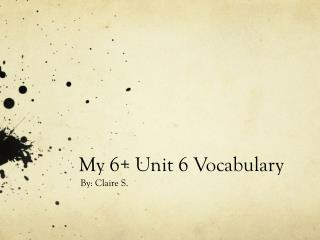DownloadDownload PresentationMy 6+ Unit 6 Vocabulary

# My 6+ Unit 6 Vocabulary

Télécharger la présentation## My 6+ Unit 6 Vocabulary

- - - - - - - - - - - - - - - - - - - - - - - - - - - E N D - - - - - - - - - - - - - - - - - - - - - - - - - - -
##### Presentation Transcript

1. My 6+ Unit 6 Vocabulary By: Claire S.

2. Constant Term • A term that doesn’t change. • 8, 88, 888, 8888, 88888, … • 3,33,333,3333,33333….

3. Division of fractions property • This is that if you divide a number, it would be the same as multiplying the same number by it’s reciprocal. • 12/2 = 12 x ½ • 14/2 = 14 x ½

4. Equation • A number sentence with an equal sign. • 13+2 = 15 • 167+1 = 168

5. Equivalent equations • Problems that have the same solution to another problem. • 6+6 = 12; 6x2 = 12 • 5+15 = 20; 5x4 = 20

6. Inequality • A number sentence with a relation symbol in it that is not equal. • 15 = 14.6 • 13< 14 /

7. Integers • A whole number and/or the opposite of that number. • 12 = -12 • 124 = -124

8. Multiplication property of -1 • Anything multiplied by -1 will become it’s OPP. • -1x 234= -234 • 8907x -1 = -8907

9. Nested parentheses • A number sentence with a set of parentheses inside of another set of parentheses. • (13+(14-2)x 6) = d • (8-(13+4)+24) = e

10. Open sentence • A number sentence that has more than 1 variable. • a+b+4= n • c+s+3=k

11. Opposite of a number • When you change a number from positive to negative, or negative to positive. Also known as OPP. • -1 = 1 • 13579 = -13579

12. Order of operations • This tells you what order to go in when solving a number sentence. • Please Excuse My Dear Aunt Sally • Parentheses, Exponents, Multiplication, Division, Addition, & Subtraction

13. Reciprocal • A number when you add it, it always is 1. • 5/3 + 3/5 • 1569/1 + 1/1569

14. Relation symbol • A symbol that shows relationship. • 7=3+4 • 5<6

15. Repeating decimals • A number in which the decimal places never end. • 0.333 • 0.161616161616161616 _ _

16. Solution • A solution to a problem is the answer. • 1x 156= 156 • 2x78= 156

17. Solution set • A set of all solutions. • J = 16 ( J=4) • G = 49 (G=7) 1 1

18. Terminating decimals • A decimal that ends, unlike Pi. • 0.7 • 0.564382

19. Trial and error method • When you try different ways to solve a problem, and you do this until you get it right, this is trial & error. • n+e = 14 ( I would have to find what n and e would be) • e+r = 19 ( I would have to find out what e and r would be)

20. Variable • A letter in a number sentence that could be any number. • 12+e=17 • 12+f=15